# Excel Array Formulas Examples

This tutorial explains how to use array formulas in real-world data problems.

Array Formulas
Array formulas are confirmed by pressing Ctrl+Shift+Enter rather than simple ENTER.

Example I

Suppose you are asked to calculate the sum of the products of column A and column B.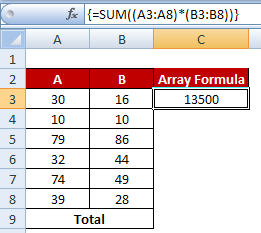Array Formula Examples
=SUM((A3:A8)*(B3:B8)) is equivalent to [(A3*B3)+ (A4*B4)+....+ (A8*B8)]
Hit CTRL SHIFT ENTER to confirm =SUM((A3:A8)*(B3:B8)). If you hit it correctly, you would see the formula wrapped in curley brackets {}.
Example II

Suppose you are asked to maximum value based on multiple criteria. The data are shown below -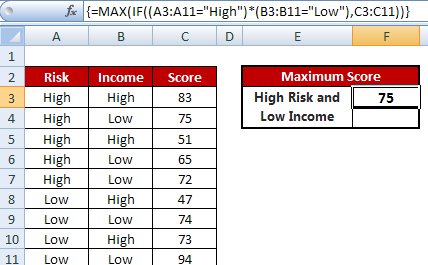Maximum Value based on Multiple Conditions
=MAX(IF((A3:A11="High")*(B3:B11="Low"),C3:C11))
Example III

Suppose you are asked to find out the top3 scorers in a class given the students can have same score.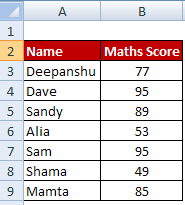Array Formulas Examples
=INDEX(\$A\$3:\$A\$9,MATCH(LARGE(\$B\$3:\$B\$9-ROW(\$B\$3:\$B\$9)/10^5,ROWS(A\$1:A1)),\$B\$3:\$B\$9-ROW(\$B\$3:\$B\$9)/10^5,0))

1. INDEX- MATCH looks for a value in the right column of a table and returns a value in the same row from a column you specify.

2. MATCH always return the first occurrence so we first need to create unique values in range.
\$B\$3:\$B\$9-ROW(\$B\$3:\$B\$9)/10^5
See how the above formula evaluates -
B3 - ROW(B3)/10^5 = 77-(3/100000)
B4 - ROW(B4)/10^5 = 95-(4/100000)
.
.
B9 - ROW(B9)/10^5 = 85-(9/100000)

It gives us unique values -
(76.99997, 94.99996,88.99995,52.99994,94.99993,48.99992,84.99991)

Example IV

We need to extract UNIQUE names from the list.Extract Unique List
Enter the following formula in cell C3
=INDEX(\$A\$3:\$A\$9,MATCH(0,COUNTIF(\$C\$2:\$C2,\$A\$3:\$A\$9),0))

Example V : Sum of Digits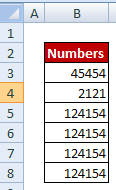Sum of Digits
=SUM(MID(B3,ROW(INDIRECT("1:" & LEN(B3))),1)*1)
The INDIRECT function returns a set of text values, in this case the values 1 through number of digits in a cell. The ROW function in turn generates a  multi-cell column array.
ROW(INDIRECT("1:" & LEN(B3)) evaluates to ROW(1:5) as the number of digits in cell B3 is 5.

Example VI : Count Uppercase and lowercase letters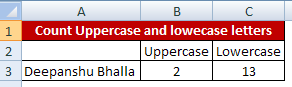Count Uppercase and Lowercase Letters
Count Uppercase letters
=SUMPRODUCT(LEN(A3)-LEN(SUBSTITUTE(A3,CHAR(ROW(INDIRECT("65:90"))),"")))
CHAR(ROW(INDIRECT("65:90"))) generates capital A through Z.

Count Lowercase letters
=SUMPRODUCT(LEN(A3)-LEN(SUBSTITUTE(A3,CHAR(ROW(INDIRECT("97:122"))),"")))
CHAR(ROW(INDIRECT("97:122"))) generates lowercase a through z.Share
Related PostsDeepanshu founded ListenData with a simple objective - Make analytics easy to understand and follow. He has over 10 years of experience in data science. During his tenure, he has worked with global clients in various domains like Banking, Insurance, Private Equity, Telecom and Human Resource.

6 Responses to "Excel Array Formulas Examples"
1.Nice post. Never tried going into array formulas but this helped a lot to understand the basics of it.

1.Thank you for stopping by my blog. Cheers!

2.thanks my name amarnathverma@outlook.com

3.Thanks. Know I have got the better undersetting of Array.

4.in example 2, why are we dividing by 10 and raise it to 5?thanks

5.INDEX(\$A\$3:\$A\$9,MATCH(LARGE(\$B\$3:\$B\$9-ROW(\$B\$3:\$B\$9)/10^5,ROWS(A\$1:A1)),\$B\$3:\$B\$9-ROW(\$B\$3:\$B\$9)/10^5,0))
Would you mind to explain 10^5 within this formula please as I am trying to understand where does it from + (A\$1:A1)) as the cells is empty

Next → ← Prev